# Strip

From 5.9 cm wide strip should be cut rhombus with area 28 cm2.

How long will be its side?

a =  4.75 cm

### Step-by-step explanation: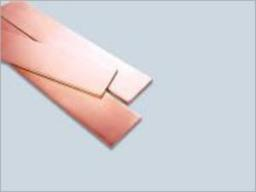Did you find an error or inaccuracy? Feel free to write us. Thank you!#### You need to know the following knowledge to solve this word math problem:

We encourage you to watch this tutorial video on this math problem:

## Related math problems and questions:

• RhombusFind the length of each side of rhombus if the perimeter is 89 cm long.
• Calculate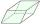Calculate the content cm2 diamond with side length 9.8 cm and a height on the side of 5 cm.
• Rhombus sidesfind the sides of a rhombus, it area is 550 cm square and altitude is 55cm
• The bookThe book has 280 pages. Each side is a rectangle with sides 15 cm and 22 cm. a) how many leaves has the book? b) at least how many square meters of paper should be used to production this book?
• Table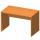Find the circumference of the table, where the long side is 1.28 meters and the short side is 86 cm.
• Rhombus constructionConstruct ABCD rhombus if its diagonal AC=9 cm and side AB = 6 cm. Inscribe a circle in it touching all sides...
• DivideDivide substance 110 cm long to two parts so that it first part is 10 cm longer than the second part and one part will be 10 times longer than the second portion. How long the parts will be?
• Tablecloth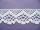The table top is 90 cm long and 50 cm wide. How many cm lace edging is necessary for the tablecloth that hangs on each side by 10 cm?
• DiamondThe diamond has a circumference of 48cm. Calculate the length of its side in dm.
• TailorTailor has an estimated 11 meters of 80 cm wide fabric. He will shape wholly 7 rectangles measuring 80 x 150 cm and nothing fabric does not remain. How many fabric he have?
• RhombusIf we increase the rhombus side by 2.4 cm; its perimeter is 20 cm. How long was the side of the rhombus?
• A butterA butter cube with an edge 6.5 cm long is packed in a package with dimensions a = 28 cm, b = 15 cm. Calculate how many cm2 the package is larger than the surface of the cube.
• Cuboid box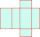Cuboid box have dimensions of 30 cm, 25 cm and 40 cm. Sketch its network and compute surface area of box.
• Perimeter from areaWhat is the perimeter of the square if its content is 64 cm2?
• Pavilion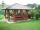The rectangular pavilion with dimensions 3.5 m and 2.75 m to be paved with square tiles of side 25 cm price of CZK 22 per 1 piece or rectangular tiles with sides of 20 cm and 15 cm in the price of CZK 11 per 1 pc. Which solution is cheaper (write its pric
• DiamondRhombus has side 17 cm and and one of diagonal 22 cm long. Calculate its area.
• Perimeter to area 2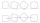Find the area of a square whose perimeter is 100 cm with solve.